# Samacheer Kalvi 6th Maths Solutions Term 1 Chapter 2 Introduction to Algebra Ex 2.1

## Tamilnadu Samacheer Kalvi 6th Maths Solutions Term 1 Chapter 2 Introduction to Algebra Ex 2.1Question 1.
Fill in the blanks:
(i) The letters a, b, c, .., x, y, z are used to represent _____
(ii) A quantity that takes _____ values is called a variable.
(iii) If there are 5 students on a bench, then the number of students in ‘n’ benches is ‘5 × n’. Here _____ is a variable.
Solution:
(i) Variables
(ii) Different
(iii) n

Question 2.
Say True or False:
(i) The length of part B in the pencil shown is ‘a – 6’.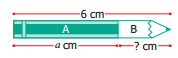(ii) If the cost of an apple is ‘x’ and cost of banana is ₹ 5, then the total cost of fruits is ₹ ‘x + 5′
(iii) If there are 11 players in a team, then there will be ’11 + q’ players in ‘q’ teams.
Solution:
(i) False
Length of B is 6 – a
(ii) True
(iii) False
There will be 11q players.

Question 3.
Draw the next two patterns and complete the table.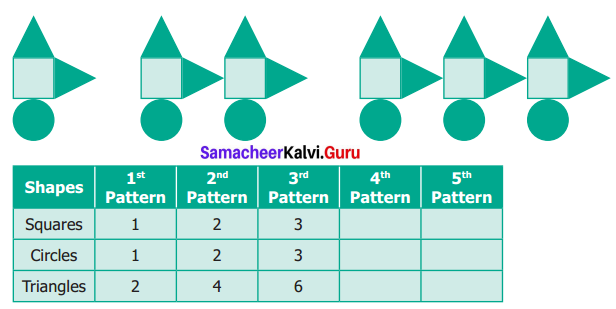Solution: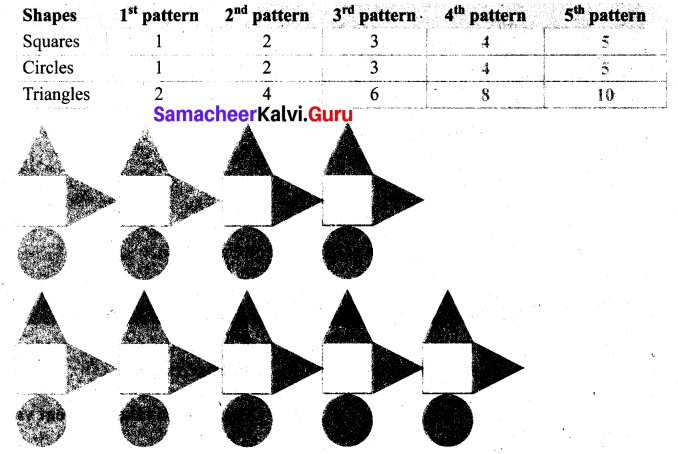Question 4.
Use a variable to write the rule, which gives the number of ice candy sticks required to make the following patterns.
(a) a pattern of letter C as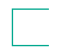(b) the pattern of letter M as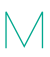Solution:
(a) A number of sticks used for 1is 3.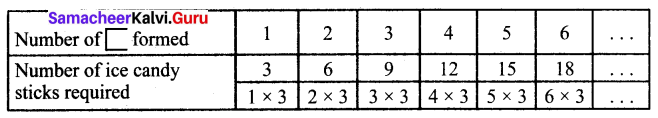If the number offormed is ‘n’ then
the number of ice candy sticks required = 3 × n = 3n.
(b) A number of sticks used for one’ is 4.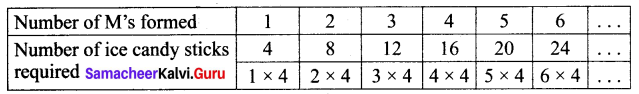The number of ice candy sticks required = 4n.

Question 5.
The teacher forms a group of five students in a class. How many students will be there in ‘p’ groups?
Solution: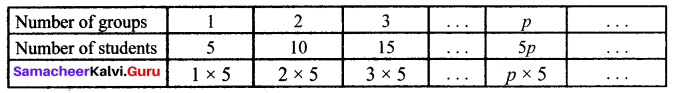5p students will be there in groups.

Question 6.
Arivazhagan is 30 years younger to his father. Write Arivazhagan’s age in terms of his father’s age.
Solution:
Let Arivazhagan’s father’s age be x years
According to the problem,
Arivazhagan’s age = (x – 30) years

Question 7.
If ‘u’ is an even number, how would you represent?
(i) the next even number?
(ii) the previous even number?
Solution:
(i) Difference between two even numbers = 2
Given that ‘u’ is an even number.
Next, even number is u + 2.
(ii) Previous even number is u – 2.Objective Type Questions

Question 8.
Variable means that it
(a) can take only a few values
(b) has a fixed value
(c) can take different values
(d) can take only 8 values
Solution:
(c) can take different values

Question 9.
‘6y’ means.
(a) 6 + y
(b) 6 – y
(c) 6 × y
(d) $$\frac{6}{y}$$
Solution:
(c) 6 × y

Question 10.
Radha is ‘x’ years of age now 4 years ago, her age was
(a) x – 4
(b) 4 – x
(c) 4 + x
(d) 4x
Solution:
(a) x – 4

Question 11.
The number of days in ‘w’ weeks is
(a) 30 + w
(b) 30w
(c) 7 + w
(d) 7w
Solution:
(d) 7wQuestion 12.
The value of ‘x’ in the circle is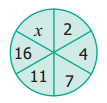(a) 6
(b) 8
(c) 21
(d) 22
Solution:
(d) 22
2 + 2 = 4, 4 + 3 = 7, 7 + 4 = 11, 11 + 5 = 16, 16 + 6 = 22